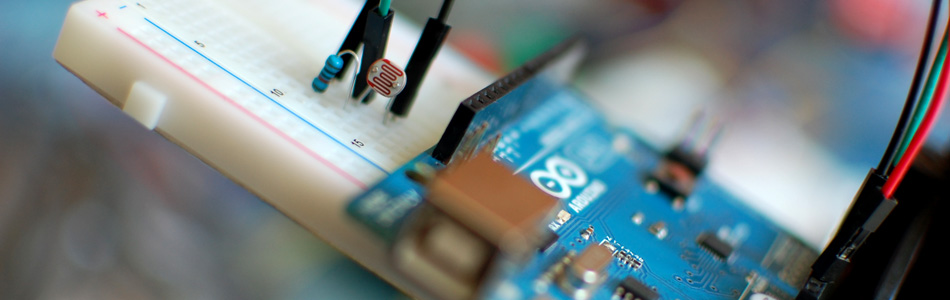The LDR (light dependent resistor) also know as the Photo-resistor (and many other things) is supposed to be day 1 of electronics. But I guess I missed the note because I never used one with my arduino maybe until now. So I guess I'm weird. But the LDR is super cheap, probably one of the easiest parts to find / use, and certainly has to have the simplest code. You can find these at any electronics store ever I imagine, or do what I did and add a few to your next sparkfun order.

If you need precise light measurement check out the TEMT6000 or the TSL230R

The LDR / Photo-resistor is basically a very simple light sensor that changes its resistance with light, lowering with more light. You can find these used in everything from the furby to automatic night lights and things like that. The LDR isn't very precise, so you cant get a quantitative LUX reading or anything like that. But it is good enough to tell the difference between light and shadow, or know if the light in your room is on/off. So if you just need to know if the light in the room has changed, or someone walked by (casting a shadow) this is your part.

Hooking it up, and why

The LDR changes its resistance with light so we can measure that change using one of the Arduino's analog pins. But to do that we need a fixed resistor (not changing) that we can use for that comparison (We are using a 10K resistor). This is called a voltage divider and divides the 5v between the LDR and the resistor. Then we measure how much voltage is on the LDR using the analog read on your arduino, and we have our reading. The amount of that 5V that each part gets is proportional to its resistance.

With the arduino analogRead, at 5V (its max) it would read 1023, and at 0v it read 0.

So if the the LDR and the resistor have the same resistance, the 5V is split evenly (2.5V), to each part. (analogRead of 512)

But if the LDR is hit with a ton of light and is reading only 1K of resistance, the 10K resistor is going to soak up 10 times as much of that 5V. So the LDR would only get .45V (analogRead of 92).

And if it is in a dark room, the LDR may be 40K or resistance, so the LDR will soak up 4 times as much of that 5V as the 10K resistor. So the LDR would get 4V (analogRead of 818).

Code

The arduino code for this just could not be easier. We are adding some serial prints and delays to it just so you can easily see the readings, but they dont need to be there if you dont need them.

int LDR_Pin = A0; //analog pin 0

void setup(){
Serial.begin(9600);
}

void loop(){